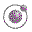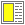Expressions, Types, and Functions - slide 8 : 46

Arithmetic expressions
Scheme uses fully parenthesized arithmetic expressions with prefix notation

Using prefix notation the operator is given before the operands

 Expression Value `(+ 4 (* 5 6))` `34` ```(define x 6) (+ (* 5 x x) (* 4 x) 3)``` `207` `(/ 21 5)` `21/5` `(/ 21.0 5)` `4.2` ```(define (fak n) (if (= n 0) 1 (* n (fak (- n 1))))) (fak 50)``` ```30414093201713378043612608166064768 844377641568960512000000000000```

There is no need for priorities - operator precedence rules - of operators in fully parenthesized expressions